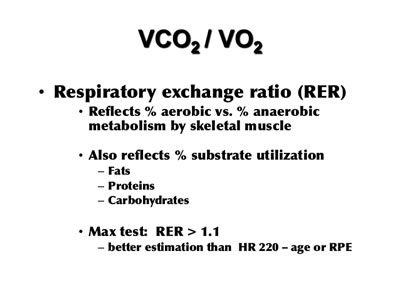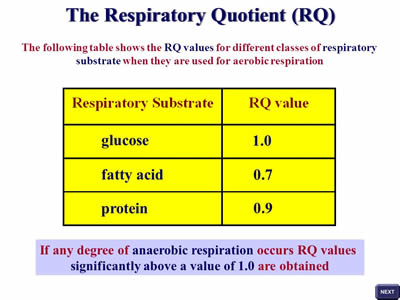# Difference between Respiratory Exchange Ratio (RER) and Respiratory Quotient (RQ)

Key Difference: RER, short for Respiratory Exchange Ratio is a ratio that aims to measure the amount of carbon dioxide (CO2) produced in comparison to the amount of oxygen (O2) used. The Respiratory Quotient (RQ), also known as respiratory coefficient, is a number that is quite useful to calculate the basal metabolic rate (BMR).Respiratory Exchange Ratio (RER) and Respiratory Quotient (RQ) are two terms that no everyone may be familiar with, but may have heard in the context of sports, fitness, or physiology, which is a part of human biology. Each human body, and in fact every living being, partakes in Respiratory Exchange Ratio (RER) and Respiratory Quotient (RQ), irrespective of whether or not they are aware of it.

The confusion between the two terms is quite common, as not only do they sound similar but are also interrelated. This dynamic is further complicated by the fact that when resting, they are in fact the same.

As its name suggests, RER, short for Respiratory Exchange Ratio is a ratio that aims to measure the amount of carbon dioxide (CO2) produced in comparison to the amount of oxygen (O2) used. Humans in general tend to inhale more oxygen than the carbon dioxide they exhale. Hence, the ratio is useful to measure the difference, which can be indicative of physical health.

As its name suggests, RER, short for Respiratory Exchange Ratio is a ratio that aims to measure the amount of carbon dioxide (CO2) produced in comparison to the amount of oxygen (O2) used. Humans in general tend to inhale more oxygen than the carbon dioxide they exhale. Hence, the ratio is useful to measure the difference, which can be indicative of physical health.

The ratio is typically measured by comparing the room air with the gases that are exhaled to find the difference. The ratio once calculated can then be quite useful for determining the Respiratory Quotient (RQ).

The Respiratory Quotient (RQ), also known as respiratory coefficient, is a number that is quite useful to calculate the basal metabolic rate (BMR). The respiratory quotient (RQ) is calculated by the formula: RQ = CO2 eliminated / O2 consumed. These days it is quite common to use an apparatus called respirometer to calculate the RQ.This is why the two are often confused, like the RER, the RQ also measures the amount of carbon dioxide (CO2) produced in comparison to the amount of oxygen (O2) used. However, while the RER calculates it as a ratio, RQ calculates it as a quotient, i.e. a number. While at first glance the two might seem the same, however, there are certain differences between the two. The RER is the ratio, dividing which one will get the RQ, which in turn is used in calculations of basal metabolic rate (BMR) when it is estimated from carbon dioxide production. Hence, it can be said that the RER can be used to calculate the RQ, which is used to calculate BMR.

Another additional difference between the two is amongst the applications of the two figures. As the RER calculates the amount of oxygen utilized by the body, in comparison to the amount of carbon dioxide expended, the RER will fluctuate depending on the amount activity. A person sitting still will have a lower ratio, as compared to someone exercising intensely, who will ideally expel larger amounts of carbon dioxide, hence resulting in a higher ratio.

Both RER and RQ also fluctuate depending on the type of substance that is being used as fuel by the body, i.e. fats, carbohydrates, protein, etc. will all yield in different ratios and quotients, which can then be used to gauge a person’s health and body composition and improve accordingly.

While, RQ can be used for dieting and fitness, the primary application of RQ is in cases of chronic obstructive pulmonary disease. Here the patients need to spend a lot of energy on respiration. By figuring out which foods result in the most RQ, the RQ can be driven down, hence resulting in conservation of energy that would have otherwise be spent on respiratory effort. This energy can then be utilized somewhere else. A RQ closer to 1 typically means more carbohydrate is being burned and a value closer to 0.7 typically means fat is being burned.

Comparison between Respiratory Exchange Ratio (RER) and Respiratory Quotient (RQ):

 Respiratory Exchange Ratio Respiratory Quotient Abbreviation RER RQ Description Is the ratio between the amount of carbon dioxide (CO2) produced in metabolism and oxygen (O2) used Is a dimensionless number used in calculations of basal metabolic rate (BMR) when estimated from carbon dioxide production. Calculates Amount of carbon dioxide (CO2) produced vs amount of oxygen (O2) used Which foods result in the most respiratory effort
```Reference: Wikipedia (RER vs RQ), TutorVista, Biology Discusion, Edward J Edmonds
Image Courtesy: slideshare.net, slideplayer.com```